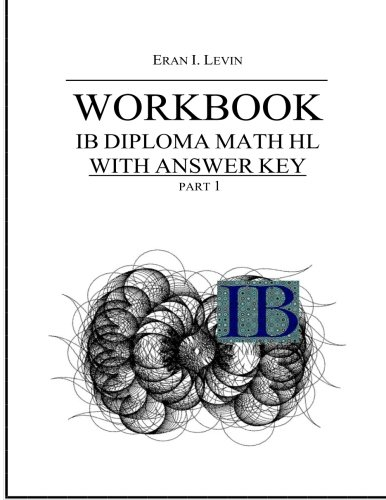## Workbook - IB Diploma Math HL part 1 with Answer Key

### Eran I. Levin

0 avg rating
( 0 ratings by Goodreads )View all copies of this ISBN edition:

This is a student workbook for the IB Math HL Diploma program More info and free material can be found at: http://ibmathworkbooks.webnode.es/ The index of the workbook is as follows: PART 1 – ALGEBRA 1.1 Types of numbers 1.2 Interval notation 1.3 Rationalization 1.4 Exponents and Logarithms 1.5 Equations 1.6 Equations with absolute value 1.7 Polynomials 1.8 Binomial Theorem 1.9 Sequences and Series 1.10 Complex numbers 1.11 Mathematical induction PART 2 – FUNCTIONS 2.1 Introduction to functions 2.2 Linear functions 2.3 Quadratic Functions 2.4 Transformations 2.5 Absolute value functions 2.6 Simple Rational functions 2.7 Exponential functions 2.8 Logarithmic functions 2.9 Radical functions 2.10 Piecewise functions 2.11 Composite functions 2.12 Inverse functions PART 3 – TRIGONOMETRY 3.1 Degrees and Radians 3.2 Definition of the Trigonometric functions 3.3 Trigonometric Identities 3.4 Trigonometric functions 3.5 Sine and Cosine Rule 3.6 Trigonometric Ratios 3.7 Inverse Trigonometric functions 3.8 Trigonometric equations 3.9 3D geometry ANSWER KEY PART 1 – ALGEBRA 1.2 Types of numbers 1.2 Interval notation 1.3 Rationalization 1.4 Exponents and Logarithms 1.5 Equations 1.6 Equations with absolute value 1.7 Polynomials 1.8 Binomial Theorem 1.9 Sequences and Series 1.10 Complex numbers 1.11 Mathematical induction PART 2 – FUNCTIONS 2.1 Introduction to functions 2.2 Linear functions 2.3 Quadratic Functions 2.4 Transformations 2.5 Absolute value functions 2.6 Simple Rational functions 2.7 Exponential functions 2.8 Logarithmic functions 2.9 Radical functions 2.10 Piecewise functions 2.11 Composite functions 2.12 Inverse functions PART 3 – TRIGONOMETRY 3.1 Degrees and Radians 3.2 Definition of the Trigonometric functions 3.3 Trigonometric Identities 3.4 Trigonometric functions 3.5 Sine and Cosine Rule 3.6 Trigonometric Ratios 3.7 Inverse Trigonometric functions 3.8 Trigonometric equations 3.9 3D geometry

"synopsis" may belong to another edition of this title.

List Price: US\$ 26.00
US\$ 42.10

Shipping: US\$ 9.71
From United Kingdom to U.S.A.

Destination, rates & speeds

## 1.Workbook - IB Diploma Math HL part 1 with Answer Key

ISBN 10: 1494923645 ISBN 13: 9781494923648
New Paperback Quantity Available: 1
Print on Demand
Seller:
Revaluation Books
(Exeter, United Kingdom)

Book Description CreateSpace Independent Publishing Platform. Paperback. Condition: Brand New. 596 pages. 11.00x8.50x1.35 inches. This item is printed on demand. Seller Inventory # zk1494923645